## 15.4.1 Control-Affine Systems

Nonholonomic system theory is restricted to a special class of nonlinear systems. The techniques of Section 15.4 utilize ideas from linear algebra. The main concepts will be formulated in terms of linear combinations of vector fields on a smooth manifold. Therefore, the formulation is restricted to control-affine systems, which were briefly introduced in Section 13.2.3. For these systems,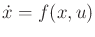is of the form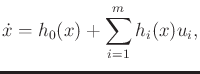(15.52)

in which each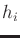is a vector field on.

The vector fields are expressed using a coordinate neighborhood of. Usually,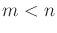, in whichis the dimension of. Unless otherwise stated, assume that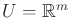. In some cases,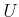may be restricted.

Each action variable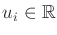can be imagined as a coefficient that determines how much of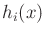is blended into the result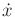. The drift term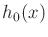always remains and is often such a nuisance that the driftless case will be the main focus. This means that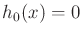for all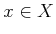, which yields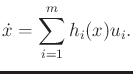(15.53)

The driftless case will be used throughout most of this section. The set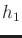,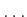,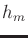, is referred to as the system vector fields. It is essential thatcontains at least an open set that contains the origin of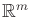. If the origin is not contained in, then the system is no longer driftless.15.7

Control-affine systems arise in many mechanical systems. Velocity constraints on the C-space frequently are of the Pfaffian form (13.5). In Section 13.1.1, it was explained that under such constraints, a configuration transition equation (13.6) can be derived that is linear if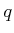is fixed. This is precisely the driftless form (15.53) using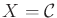. Most of the models in Section 13.1.2 can be expressed in this form. The Pfaffian constraints on configuration are often called kinematic constraints because they arise due to the kinematics of bodies in contact, such as a wheel rolling. The more general case of (15.52) for a phase spacearises from dynamic constraints that are obtained from Euler-Lagrange equation (13.118) or Hamilton's equations (13.198) in the formulation of the mechanics. These constraints capture conservation laws, and the drift term usually appears due to momentum.

Example 15..5 (A Simplified Model for Differential Drives and Cars)   Both the simple-car and the differential-drive models of Section 13.1.2 can be expressed in the form (15.53) after making simplifications. The simplified model, (15.48), can be adapted to conveniently express versions of both of them by using different restrictions to define. The third equation of (15.48) can be reduced towithout affecting the set of velocities that can be achieved. To conform to (15.53), the equations can then be written in a linear-algebra form as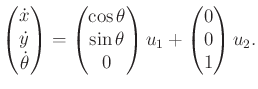(15.54)

This makes it clear that there are two system vector fields, which can be combined by selecting the scalar values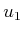and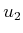. One vector field allows pure translation, and the other allows pure rotation. Without restrictions on, this system behaves like a differential drive because the simple car cannot execute pure rotation. Simulating the simple car with (15.54) requires restrictions on(such as requiring thatbeor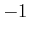, as in Section 15.3.2). This is equivalent to the unicycle from Figure 13.5 and (13.18).

Note that (15.54) can equivalently be expressed as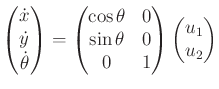(15.55)

by organizing the vector fields into a matrix.In (15.54), the vector fields were written as column vectors that combine linearly using action variables. This suggested that control-affine systems can be alternatively expressed using matrix multiplication in (15.55). In general, the vector fields can be organized into an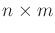matrix as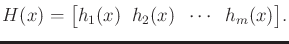(15.56)

In the driftless case, this yields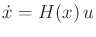(15.57)

as an equivalent way to express (15.53)

It is sometimes convenient to work with Pfaffian constraints,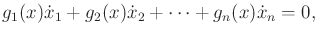(15.58)

instead of a state transition equation. As indicated in Section 13.1.1, a set ofindependent Pfaffian constraints can be converted into a state transition equation with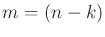action variables. The resulting state transition equation is a driftless control-affine system. Thus, Pfaffian constraints provide a dual way of specifying driftless control-affine systems. ThePfaffian constraints can be expressed in matrix form as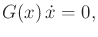(15.59)

which is the dual of (15.57), and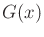is a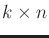matrix formed from the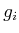coefficients of each Pfaffian constraint. Systems with drift can be expressed in a Pfaffian-like form by constraints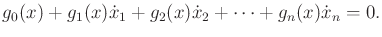(15.60)

Steven M LaValle 2020-08-14